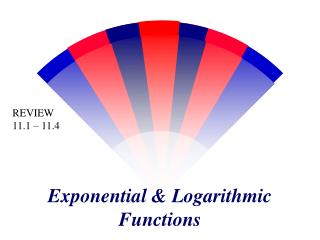DownloadDownload PresentationExponential & Logarithmic Functions

# Exponential & Logarithmic Functions

Télécharger la présentation## Exponential & Logarithmic Functions

- - - - - - - - - - - - - - - - - - - - - - - - - - - E N D - - - - - - - - - - - - - - - - - - - - - - - - - - -
##### Presentation Transcript

1. REVIEW 11.1 – 11.4 Exponential & Logarithmic Functions

2. Evaluate: 1.) 2.) 3.) 4.) 5.) 6.) 7.) 8.) 9.) 10.) 11.) 12.)

3. Express using rational exponents: 1.) 2.) 3.) 4.) 5.) 6.) 7.) 8.) 9.) 10.) 11.) 12.)

4. Express using radicals: 1.) 2.) 3.) 4.) 5.) 6.) 7.) 8.) 9.) 10.) 11.) 12.)

5. Simplify: 1.) 2.) 3.) 4.) 5.) 6.) 7.) 8.) 9.) 10.) 11.) 12.)

6. General Form of Exponential Function y = b x where b > 1 • Domain: All reals • Range: y > 0 • x-intercept: None • y-intercept: (0, 1)

7. General Form of Exponential Functiony = b (x + h) + kwhere b > 1 • h moves graph left or right (opposite way) • k move graph up or down (expected way) • So y=3(x+2) + 3 moves the graph 2 units to the left and 3 units up • (0, 1) to (– 2, 4)

8. Graph: 9 3 1 1/3 0.111 0.037 Decreasing for all of x

9. Graph: 0.125 0.25 0.5 1 2 4 Increasing for all of x

10. Graph: -0.5 -1 -2 -4 -8 -16 Decreasing for all of x

11. Graph: 8 4 2 1 0.5 0.25 Decreasing for all of x

12. Graph: 0.25 0.5 1 2 4 8

13. Graph: 4 2 1 0.5 0.25 0.125

14. Converting between Exponents & Logarithms WRITE EACH EQUATION INTO A LOG FUNCTION: WRITE EACH EQUATION INTO A EXPONENTIAL FUNCTION:

15. Evaluate: Evaluate: Evaluate: 3 -3 6 Evaluate: Evaluate: Evaluate: -4 1 256

16. Properties of Logarithms (Shortcuts) • logb1 = 0 (because b0 = 1) • logbb = 1 (because b1 = b) • logb(br)= r (because br = br) • blog b M = M (because logbM= logbM)

17. logb(MN)= logbM + logbN Ex: log4(15)= log45 + log43 logb(M/N)= logbM – logbN Ex: log3(50/2)= log350 – log32 logbMr = r logbM Ex: log7 (103) = 3 log7 10 If logbM = logbN Then M = N log11 (1/8) = log11 8-1 Properties of Logarithms

18. TRY THESE TO SOLVE THESE: First I would change to exponential form!!

19. It appears that we have 2 solutions here. If we take a closer look at the definition of a logarithm however, we will see that not only must we use positive bases, but also we see that the arguments must be positive as well. Therefore -2 is not a solution.

20. Our final concern then is to determine why logarithms like the one below are undefined. Can anyone give us an explanation ? One easy explanation is to simply rewrite this logarithm in exponential form. We’ll then see why a negative value is not permitted. What power of 2 would gives us -8 ? Hence expressions of this type are undefined.

21. SOLVE EACH LOGARITHM EQUATION: 1.) 2.) 3.) 4.)

22. Same Base • Solve: 4x-2 = 64x • 4x-2 = (43)x • 4x-2 = 43x • x–2 = 3x • -2 = 2x • -1 = x 64 = 43 If bM = bN, then M = N If the bases are already =, just solve the exponents

23. You Do • Solve 27x+3 = 9x-1

24. Review – Change Logs to Exponents 32 = x, x = 9 • log3x = 2 • logx16 = 2 • log 1000 = x x2 = 16, x = 4 10x = 1000, x = 3

25. Example • 7xlog25 = 3xlog25 + ½ log225 • log257x = log253x + log225 ½ • log257x = log253x + log251 • 7x = 3x + 1 • 4x = 1

26. Example 1 – Solving Simple Equations • a. 2x= 32 2x= 25x = 5 • b. ln x – ln 3 = 0 ln x = ln 3 x = 3 • c. = 9 3–x= 32 x = –2 • d. ex= 7 ln ex= ln 7 x = ln 7 • e. ln x = –3 eln x= e–3 x = e–3 • f. log x =–1 10log x = 10–1x = 10–1 = • g. log3x = 4 3log3x = 34x = 81

27. Example 6 – Solving Logarithmic Equations • a. ln x = 2 • eln x= e2 • x = e2 • b. log3(5x – 1) = log3(x + 7) • 5x – 1 = x + 7 • 4x = 8 • x = 2

28. Example 6 – Solving Logarithmic Equations cont’d • c. log6(3x + 14) – log6 5 = log62x • 3x + 14 = 10x • –7x = –14 • x = 2

29. Given the original principal, the annual interest rate, and the amount of time for each investment, and the type of compounded interest, find the amount at the end of the investment. 1.) P = \$1,250; r = 8.5%; t = 3 years; quarterly 2.) P = \$2,575; r = 6.25%; t = 5 years, 3 months; continuously